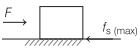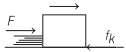# What is Friction in Physics? | Definition, Examples, Types of Friction – Laws of Motion

Friction Definition Physics:
A force acting on the point of contact of the objects, which opposes the relative motion is called friction.

## What is Friction in Physics? | Definition, Examples, Types of Friction – Laws of Motion

We are giving a detailed and clear sheet on all Physics Notes that are very useful to understand the Basic Physics Concepts.

• It acts parallel to the contact surfaces.
• Frictional forces are produced due to intermolecular interactions acting between the molecules of the bodies in contact.

Types of Friction:

1. Static Friction
2. Limiting Friction
3. Kinetic Friction

1. Static Friction Definition:
It is an opposing force which comes into play when one body tends to move over the surface of the other body but actual motion is not taking place.

Static friction is a self-adjusting force which increases as the applied force is increased. Static friction opposes impending motion.

2. Limiting Friction Definition:
It is the maximum value of static friction when body is at the verge of starting motion.

Limiting friction, fs(max) = μlR

where, μl = coefficient of limiting friction and R = normal reaction.
Limiting friction do not depend on area of contact surfaces but depends on their nature, i.e. smoothness or roughness.Angle of Friction Definition:
It is the angle which the resultant of the force of limiting friction and the normal reaction(N) makes with the direction of N.

μl = tan θ

Angle of Repose in Friction or Angle of Sliding
It is the minimum angle of inclination of a plane with the horizontal, such that a body placed on it, just begins to slide down.
If angle of repose is a and coefficient of limiting friction is μl, then

μl = tan α

3. Kinetic Friction Definition:
It is an opposing force that comes into existence when one object is actually moving over the surface of other object.Kinetic friction (fk) = μk R

where, μk = coefficient of kinetic friction and R = normal reaction.

Kinetic friction is of two types:
(a) Sliding friction
(b) Rolling friction
As, rolling friction < sliding friction, therefore it is easier to roll a body than to slide.

Laws of Motion:
There are various laws in Physics that define the motion of the object. When an object is in motion whether it is linear or circular there is some force which is always imposed on it.